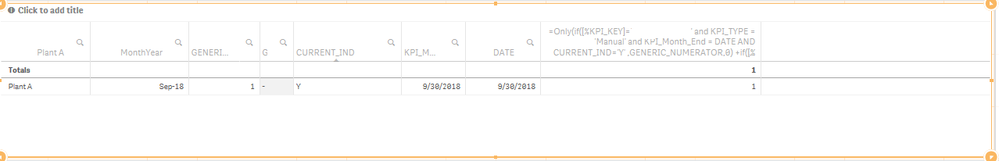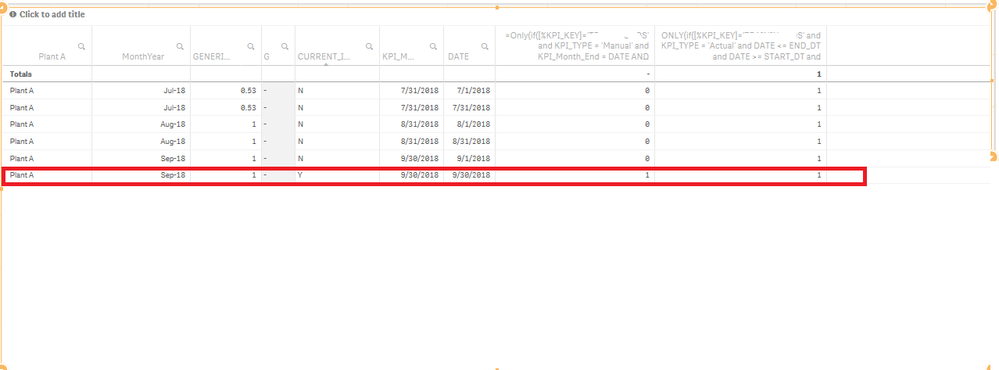# Qlik Sense App Development

Announcements
Coming your way, the Qlik Data Revolution Virtual Summit. October 27-29. REGISTER
cancel
Showing results for
Did you mean:
HighlightedCreator II

## ONLY() errors out in #1KPI and Gauge

Hi ,

I am using  below expression in Charts and it works fine as expected ,  but if I use this expression in #1KPI it returns nothing . and for gauge it returns me the correct numbers but wont let me set gauge colors .

ONLY(if([%KPI_PLANT]='A' and KPI_TYPE = 'Manual' and KPI_Month_End = DATE AND Ind='Y',Generic_NUM,0)
+if([%KPI_PLANT]='A' and KPI_TYPE = 'Actual' and DATE <= END_DT and DATE >= START_DT,Generic_NUM,0))
/
ONLY(if([%KPI_PLANT]='A' and KPI_TYPE = 'Actual' and DATE <= END_DT and DATE >= START_DT and ORD_DEN >0,ORD_DEN, 1))

Any Thoughts why  only() works differently and how I can make it work  ?

Labels (1)
• ### Qlik Community

7 Replies
HighlightedMVP

Do you get multiple rows in the chart where this works? and are those values different?

HighlightedCreator IINo I get single the record .

HighlightedMVP

Can you try them as two separate expressions in the chart and see if you still see a single row for both the expressions?

```ONLY(if([%KPI_PLANT]='A' and KPI_TYPE = 'Manual' and KPI_Month_End = DATE AND Ind='Y',Generic_NUM,0)
+if([%KPI_PLANT]='A' and KPI_TYPE = 'Actual' and DATE <= END_DT and DATE >= START_DT,Generic_NUM,0))```
`ONLY(if([%KPI_PLANT]='A' and KPI_TYPE = 'Actual' and DATE <= END_DT and DATE >= START_DT and ORD_DEN >0,ORD_DEN, 1))`

HighlightedCreator II

Hi Sunny

here is the out come . I need to show only last row in my KPI & Gauge.HighlightedMVP

So, you numerator seems to be the problem here... may be you can try one of these

```Max(If([%KPI_PLANT] = 'A' and KPI_TYPE = 'Manual' and KPI_Month_End = DATE and Ind = 'Y', Generic_NUM, 0)
+If([%KPI_PLANT] = 'A' and KPI_TYPE = 'Actual' and DATE <= END_DT and DATE >= START_DT, Generic_NUM, 0))
/
Only(If([%KPI_PLANT] = 'A' and KPI_TYPE = 'Actual' and DATE <= END_DT and DATE >= START_DT and ORD_DEN > 0, ORD_DEN, 1))```

or

```Sum(if([%KPI_PLANT]='A' and KPI_TYPE = 'Manual' and KPI_Month_End = DATE AND Ind='Y',Generic_NUM,0)
+if([%KPI_PLANT]='A' and KPI_TYPE = 'Actual' and DATE <= END_DT and DATE >= START_DT,Generic_NUM,0))
/
ONLY(if([%KPI_PLANT]='A' and KPI_TYPE = 'Actual' and DATE <= END_DT and DATE >= START_DT and ORD_DEN >0,ORD_DEN, 1))```
HighlightedCreator II
TY So Much Sunny( my virtual guru :P) . It did work , I see where I was going wrong . I tried sum before but I used in both numerator and denominator and that didn't help.

Till Next Happy Holidays & a very Happy New Year!!!

HighlightedMVP

Thank you and same to you!!

Best,
Sunny